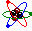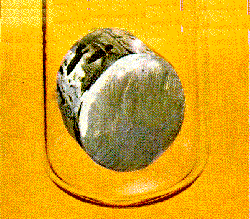##Abbreviations for the elements in ChemistryThe Rutherford model of the atom; the element ytterbium.1

### David Bar-Tzur

Created 21 June 2001

Print this table out and use it during Chemistry class to look up abbreviations you can use for the elements being discussed.

 actinium = Ac aluminum = Al amercium = Am antimony = Sb argon = Ar arsenic = As astatine = At barium = Ba berkelium = Bk beryllium = Be bismuth = Bi bohrium = Bh boron = B bromine = Br cadmium = Cd calcium = Ca californium = Cf carbon = C cerium = Ce cesium = Cs chlorine = Cl chromium = Cr cobalt = Co copper = Cu curium = Cm dubnium = Db dysprosium = Dy einsteinum = Es erbium = Er europium = Eu fermium = Fm fluorine = F francium = Fr gadolinium = Gd gallium = Ga germanium = Ge gold = Au hafnium = Hf hahnium = Hn helium = He holmium = Ho hydrogen = H indium = In iodine = I iridium = Ir iron = Fe juliotium = Jl krypton = Kr lanthanum = La lawrencium = Lr lead = Pb lithium = Li lutetium = Lu magnesium = Mg manganese = Mn meltnerium = Mt mendelevium = Md mercury = Hg molybdenum = Mo neodymium = Nd neon = Ne neptunium = Np nickel = Ni niobium = Nb nitrogen = N nobelium = No osmium = Os oxygen = O palladium = Pd phosphorus = P platinum = Pt plutonium = Pu polonium = Po potassium = K prasodymium = Pr promethium = Pm protactinium = Pa radium = Ra radon = Rn rhodium = Rh rubidium = Rb ruthenium = Ru rutherfordium = Rf rhenium = Re samarium = Sm scandium = Sc selenium = Se silicon = Si silver = Ag sodium = Na strontium = Sr sulphur = S technetium = Tc tantalum = Ta tellurium = Te terbium = Tb thallium = Tl thorium = Th thulium = Tm tin = Sn titanium = Ti tungsten = W uranium = U vanadium = V xenon = Xe ytterbium = Yb yttrium = Y zinc = Zn zirconium = Zr

The names of the heavy elements whose atomic numbers are > 100 are formed by using affixes for each digit as follows: 0 = nil (n), 1 = un (u), 2 = bi (b), 3 = tri (t), 4 = quad (q), 5 = pent (p), 6 = hex (h), 7 = sept (s), 8 = oct (o), 9 = enn or en if it's the last digit (e) + "ium" is added at the end. For example, element 104 is unnilquadium (Unq), element 105 is Unnilpentium (Unp), and so on. The old names for 100-103 are still acceptible. And for fun, listen to Tom Lehrer singing the "Song of the elements" at Elements.swf.

Image credits

1. On the left (Rutherford model of the atom): from ClipArtConnection.com and on the right (sample of ytterbium): from http://nobel.scas.bcit.bc.ca/resource/ptable/yb.htm (Ytterbium), which is no longer extant.

Home